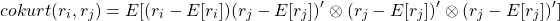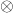# Curse of dimensionality part 3: Higher-Order Comoments

Higher moments such as Skewness and Kurtosis are not as explored as they should be.

These moments are crucial for managing portfolio risk. At least as important as volatility, if not more. Skewness relates to asymmetry risk and Kurtosis relates to tail risk.

Despite their great importance, those higher moments enjoy only a small portion of attention compared with their lower more friendly moments: the mean and the variance. In my opinion, one reason for this may be the impossibility of estimating those moments, estimating them accurately that is.

It is yet another situation where Curse of Dimensonality rears its enchanting head (and an idea for a post is born..).

Mean variance portfolio optimization is center-stage. But there is much more in the way a portfolio of assets behaves. How the assets move around together, the co-movement of the assets.

Sure, the most important is the vector of means – the levels. Second is the covariance matrix, how tightly the assets move together in terms of variability. Low values populating the covariance matrix means that we are variance-diversified: a sharp move of only one asset does not have very big impact on the portfolio as a whole, we like that. But What about coskewness and cokurtosis? What a sharp move in one of the assets means for the total portfolio in terms of skewness, or in terms of kurtosis? Most strategies don’t like heavy tails returns distribution for example.

When building a portfolio we usually disregard higher moments. Put differently, we assume the assets are higher-moments independent. If we want to relax this assumption a very familiar tradeoff emerges: [added estimation noise] vs [better portfolio weights]. How much more estimation noise, how much better are the weights? By way of contradiction, if the weights are not changing much, the added estimation noise and estimation efforts are not worth it.

Harvey Campbell and colleagues (2010) investigated how influential is skewness in terms of finding optimal portfolio weights. I am not aware of any work trying something similar for kurtosis. Perhaps for a good reason. This is one of the most barbed research topics I have seen, so quite risky from a research-career perspective.

A matrix is also a second order tensor. A matrix is one dimension higher than a vector, which is a first-order tensor (a scalar can be thought of as a zero-order tensor). A third order tensor is a cube, or an array with one dimension higher than a matrix. This is what comes after the covariance matrix, a coskewness cube. A forth order tensor is called a Tesseract (yeah, that blue thingy from “Thor” movie), and that is a beastly beast to tame, estimation-wise.

Mathematically it does not look like much:The first line appears in all basic probability courses. The second and third lines are straight-forward extensions. The problem is theoperator you see there. This candy is called the Kronecker product. Apply this operator between say a vector A and a matrix B, each element of the vector now expands itself to become a matrix in its own right, with the same dimension as matrix B. Simply speaking each element in A is multiplied by all the elements in B. So the word “expand” is apt here.

Denote n as the number of assets in the portfolio. Due to symmetry, a covariance matrix has “only”unique entries. Already quite a large number of parameters, and so quite some estimation noise. Massive number of papers sprung tackling this problem. Honey, I Shrunk the Sample Covariance Matrix to mention one favorite. For coskewness we have, and for cokurtosis we have. Intimidating, but not all hope is lost.

The paper “Improved estimates of higher-order comoments and implications for portfolio selection” introduces improved estimators for those comoments of asset returns. Think about it as a direct extension of the solutions applied to the covariance matrix. Shrinkage, factor modelling etc. Yes, because there are many more parameters those techniques should be applied more forcefully. Single-factor approach seems to work quite well.

Below some R-code for estimating comoments, and demonstrating the inflation of parameters when we up the dimension. Below that there are some references for papers mentioned in this post.

So for 4 assets the cokurtosis matrix has dimension of. This kind of expansion pretty much closes the lid on working with single names, but perhaps relevant for cross-assets portfolios.

## References

• Nice Introduction to Skewness by Jonathan Regenstein.
• Lionel Martellini and Volker Ziemann. Improved estimates of higher-order comoments and implications for portfolio selection. The Review of Financial Studies, 23(4):1467–1502, 2009
• Campbell R Harvey, John C Liechty, MerrillWLiechty, and Peter Müller. Portfolio selection with higher moments. Quantitative Finance, 10(5):469–485, 2010